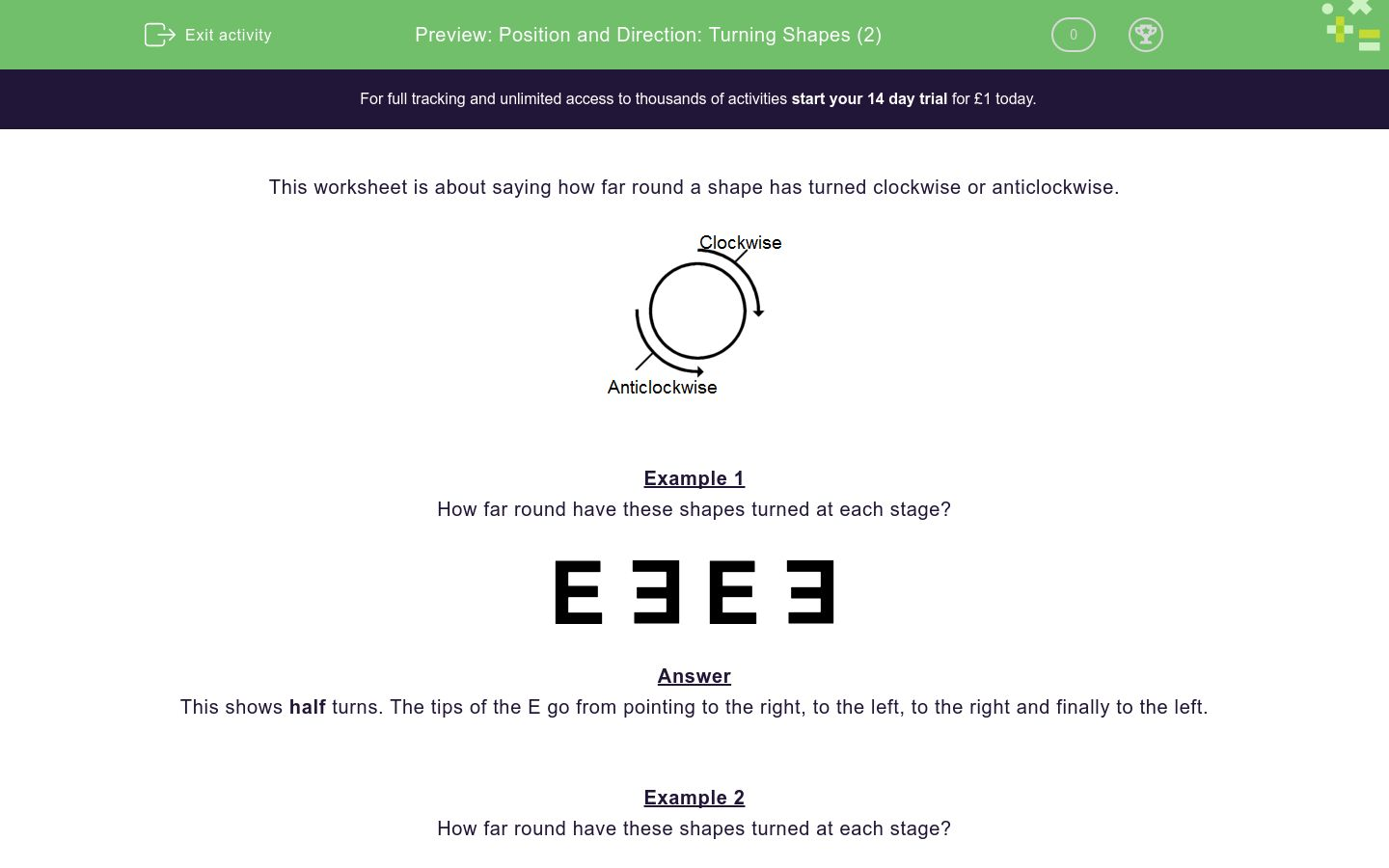# Position and Direction: Turning Shapes (2)

In this worksheet, students spot quarter and half turns, clockwise and anticlockwise.Key stage:  KS 1

Curriculum topic:   Geometry: Position and Direction

Curriculum subtopic:   Use Language of Position/Direction

Difficulty level:### QUESTION 1 of 10

This worksheet is about saying how far round a shape has turned clockwise or anticlockwise.Example 1

How far round have these shapes turned at each stage?This shows half turns. The tips of the E go from pointing to the right, to the left, to the right and finally to the left.

Example 2

How far round have these shapes turned at each stage?This shows quarter clockwise turns.

Look at the tip of the arrow. Imagine a clock face.

The tip goes from 12 to 3 to 6 to 9. At each stage it is moving clockwise a quarter turn.

How far round have these shapes turned at each stage?quarter clockwise

quarter anticlockwise

half

How far round have these shapes turned at each stage?quarter clockwise

quarter anticlockwise

half

How far round have these shapes turned at each stage?quarter clockwise

quarter anticlockwise

half

How far round have these shapes turned at each stage?quarter clockwise

quarter anticlockwise

half

How far round have these shapes turned at each stage?quarter clockwise

quarter anticlockwise

half

How far round have these shapes turned at each stage?quarter clockwise

quarter anticlockwise

half

How far round have these shapes turned at each stage?quarter clockwise

quarter anticlockwise

half

How far round have these shapes turned at each stage?quarter clockwise

quarter anticlockwise

half

How far round have these shapes turned at each stage?quarter clockwise

quarter anticlockwise

half

How far round have these shapes turned at each stage?quarter clockwise

quarter anticlockwise

half

• Question 1

How far round have these shapes turned at each stage?quarter clockwise
EDDIE SAYS
Look at the black part, which is moving a quarter turn clockwise at each stage.
• Question 2

How far round have these shapes turned at each stage?quarter anticlockwise
EDDIE SAYS
Look at the tip of the arrow, which is moving a quarter turn anticlockwise at each stage.
• Question 3

How far round have these shapes turned at each stage?half
EDDIE SAYS
Look at the top of the A, which is moving up and down at each stage, i.e. half turns.
• Question 4

How far round have these shapes turned at each stage?quarter clockwise
EDDIE SAYS
Look at the tip of the arrow, which is moving a quarter turn clockwise at each stage.
• Question 5

How far round have these shapes turned at each stage?half
EDDIE SAYS
Look at the tips of the E, which are moving right to left, i.e. half turns.
• Question 6

How far round have these shapes turned at each stage?quarter anticlockwise
EDDIE SAYS
Look at the black part, which is moving a quarter turn anticlockwise at each stage.
• Question 7

How far round have these shapes turned at each stage?half
EDDIE SAYS
Look at the tips of the arrows, which are up then down etc., i.e. half turns.
• Question 8

How far round have these shapes turned at each stage?half
EDDIE SAYS
Look at the black parts, which are up then down etc., i.e. half turns.
• Question 9

How far round have these shapes turned at each stage?half
EDDIE SAYS
These are also half turns, because the black parts go right, left, right, left.
• Question 10

How far round have these shapes turned at each stage?half
EDDIE SAYS
Again half turns because the tips of the arrow go left, right, left, right.
---- OR ----

Sign up for a £1 trial so you can track and measure your child's progress on this activity.

### What is EdPlace?

We're your National Curriculum aligned online education content provider helping each child succeed in English, maths and science from year 1 to GCSE. With an EdPlace account you’ll be able to track and measure progress, helping each child achieve their best. We build confidence and attainment by personalising each child’s learning at a level that suits them.

Get started